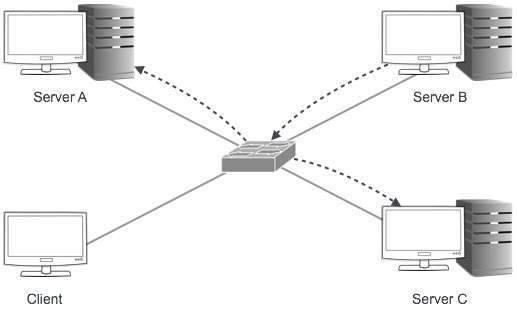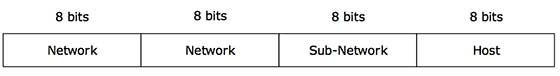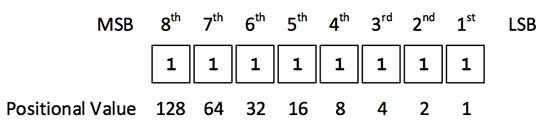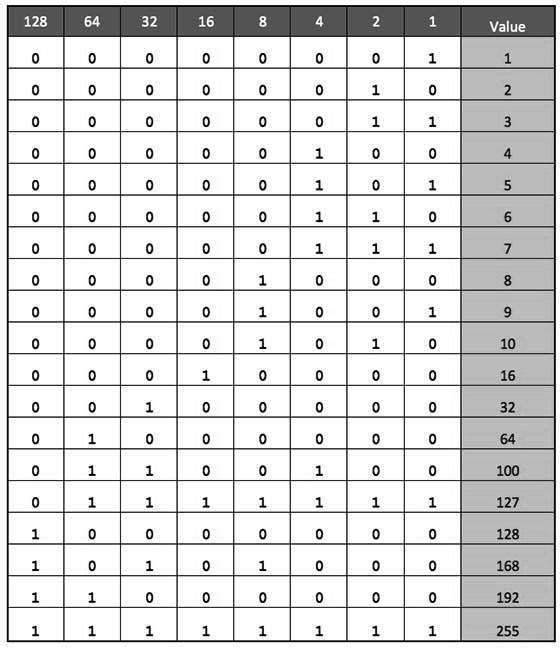IPv4 supports three different types of addressing modes. −

In this mode, data is sent only to one destined host. The Destination Address field contains 32- bit IP address of the destination host. Here the client sends data to the targeted server −In this mode, the packet is addressed to all the hosts in a network segment. The Destination Address field contains a special broadcast address, i.e. 255.255.255.255. When a host sees this packet on the network, it is bound to process it. Here the client sends a packet, which is entertained by all the Servers −This mode is a mix of the previous two modes, i.e. the packet sent is neither destined to a single host nor all the hosts on the segment. In this packet, the Destination Address contains a special address which starts with 224.x.x.x and can be entertained by more than one host.Here a server sends packets which are entertained by more than one servers. Every network has one IP address reserved for the Network Number which represents the network and one IP address reserved for the Broadcast Address, which represents all the hosts in that network.

IPv4 uses hierarchical addressing scheme. An IP address, which is 32-bits in length, is divided into two or three parts as depicted −A single IP address can contain information about the network and its sub-network and ultimately the host. This scheme enables the IP Address to be hierarchical where a network can have many sub-networks which in turn can have many hosts.This way the Subnet Mask helps extract the Network ID and the Host from an IP Address. It can be identified now that 192.168.1.0 is the Network number and 192.168.1.152 is the host on that network.

## Binary Representation

The positional value method is the simplest form of converting binary from decimal value. IP address is 32 bit value which is divided into 4 octets. A binary octet contains 8 bits and the value of each bit can be determined by the position of bit value '1' in the octet.Positional value of bits is determined by 2 raised to power (position – 1), that is the value of a bit 1 at position 6 is 2^(6-1) that is 2^5 that is 32. The total value of the octet is determined by adding up the positional value of bits. The value of 11000000 is 128+64 = 192. Some examples are shown in the table below −